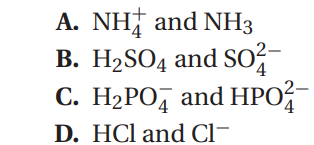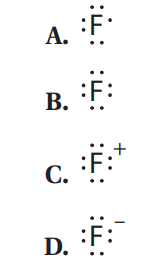# MCAT Chemistry Practice Test 2023 (59 Questions Answers)

MCAT Chemistry Practice Test 2023 (59 Questions Answers): Association of American Medical Colleges (AAMC) Medical College Admission Test (MCAT) Chemical and Physical Foundations of Biological Systems practice test questions answers. In addition, you can download a printable copy of the PDF for better MCAT Test Prep & Review.

The MCAT Chemistry Practice Test questions are designed to match the real exam as closely as possible in format and degree of difficulty. If you approach these tests as you would the real exam, your results will give you a good idea of how well you would score if you took the real MCAT Chemistry test today.

## MCAT Chemistry Practice Test 2023

 Test Name MCAT Practice Test 2023 Subjects Chemical and Physical Foundations of Biological Systems Printable PDF N/A Questions Format Multiple Choice Question Total passage-based sets of questions 10 sets 4-6 questions per set Total independent questions 15 Total Questions 59 Questions Time Limit: 95 Minutes

Passage 1

Many alkenes can be hydrated simply by treatment with a strong acid in the presence of water. The limitation of this method is that a carbocation is generated along the mechanistic pathway, opening the door to rearrangements, as shown in the sulfuric acid–catalyzed hydration of 3,3-dimethylbut-1-ene here:One workaround for this problem is the oxymercuration-demercuration sequence, as shown here: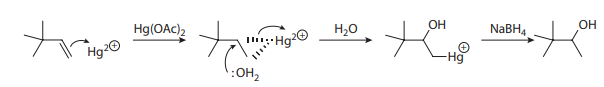Consider the hydration of 3,6-dimethylcyclohex-1-ene under two separate conditions, for which four different alcohol products are proposed: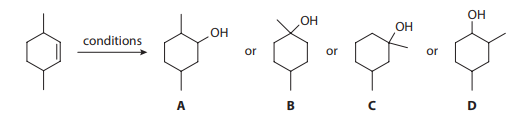Q1. The most reasonable major hydration product of 3,6-dimethylcyclohex-1-ene using water and sulfuric acid is:

• A. Product A
• B. Product B
• C. Product C
• D. Product D

Q2. The most reasonable major hydration product of 3,6-dimethylcyclohex-1-ene using oxymercuration-demercuration is:

• A. Product A
• B. Product B
• C. Product C
• D. Product D

Q3. The structure giving six unique 13C-NMR signals is most consistent with:

• A. Product A
• B. Product B
• C. Product C
• D. Product D

Q4. Which of the following statements is MOST true for trans-3,6-dimethylcyclohex1-ene?

• A. There are two chiral centers and the molecule is chiral.
• B. There are two chiral centers and the molecule is achiral.
• C. There are no chiral centers and the molecule is chiral.
• D. There are no chiral centers and the molecule is achiral.

Q5. The by-product of demercuration is:

• A. Hg0
• B. Hg+
• C. Hg2+
• D. Hg3+

Questions 6–8 are not associated with a passage.

Q6. A peregrine falcon of 9 N is a bird known for its ability to reach extremely high speeds as it pursues prey. A bird in flight is subject to four forces: weight, drag, lift, and thrust. Which free body diagram accurately illustrates the direction of the forces acting on the bird assuming the bird is moving at constant velocity?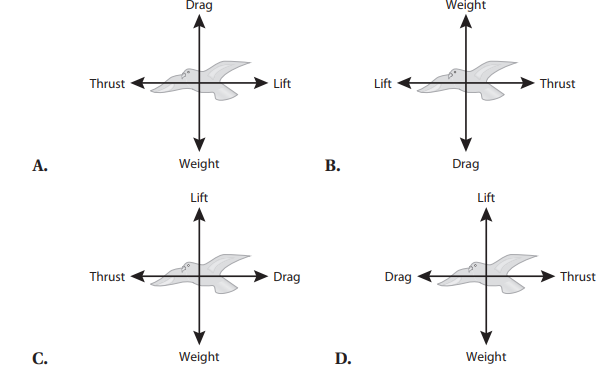Q7. Blood is pumped from the heart at a rate of 5 L/min into the aorta of a typical radius 1.2 cm. The velocity of blood as it enters the aorta is MOST nearly:

• A. 9 \frac{cm}{s}
• B. 12  \frac{cm}{s}
• C. 18 \frac{cm}{s}
• D. 32 \frac{cm}{s}

Q8. In response to a heart attack, the normal heart rhythm is restored by a cardiac defibrillator, which transmits approximately 15 amperes of current through the chest during a time interval of 7 milliseconds. The amount of charge that flows during that time is:

• A. 0.1 C
• B. 0.6 C
• C. 1.0 C
• D. 1.2 C

Q8. In response to a heart attack, the normal heart rhythm is restored by a cardiac defibrillator, which transmits approximately 15 amperes of current through the chest during a time interval of 7 milliseconds. The amount of charge that flows during that time is:

• A. 0.1 C
• B. 0.6 C
• C. 1.0 C
• D. 1.2 C

Passage 2

The reaction between sodium metal and chlorine gas is spontaneous and releases energy. The energy that is released is the enthalpy of formation of solid sodium chloride, which is also called the heat of formation. The reaction takes place in five elementary steps:

1. Sodium is vaporized.
Na (s) → Na (g )                                                     ΔHorxn = 92 kJ

2. Chlorine decomposes into atoms.
\frac{1}{2} Cl2 (g ) → Cl (g )      ΔHorxn = 121 kJ

3. Gaseous sodium atoms ionize.
Na (g ) → Na+ (g )+ e                                        ΔHorxn = 496 kJ
This quantity is the first ionization energy of sodium vapor.

4. The electron adds to a chlorine atom.
Cl (g ) + e → Cl (g )                                          ΔHorxn = −349 kJ
This quantity is the electron affinity of chlorine.

5. The chloride ion reacts with the sodium ion.
Cl (g ) + Na+ (g ) → NaCl (s)                        ΔHorxn = −771 kJ

Addition of the five elementary equations gives the sum equation for the formation of solid sodium chloride. Most of the energy that is given off is due to the formation of solid sodium chloride from the gaseous ions. In solid sodium chloride, each positive ion is surrounded by 6 negative ions, and each negative ion is surrounded by 6 positive ions in a cubic lattice array. The lattice energy of an ionic compound is a measure of the attractive forces between the ions. The higher the lattice energy, the stronger the attractions and the higher melting the solid. Lattice energies depend on the charge and on the size of the ions in the array. If the size is constant, increasing charge increases the lattice energy. If the charge is constant, increasing size lowers the lattice energy.

Some lattice energies are given in the table.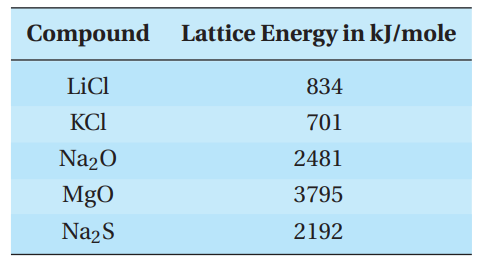Q9. When 1 mole of sodium metal reacts with 1 mole of chlorine gas to form solid sodium chloride, what is the heat of formation?

• A. −771 kJ
• B. +771 kJ
• C. −411 kJ
• D. +411 kJ

Q10. What is the electron configuration of chloride?

• A. 1s²2s²2p63s23p5
• B. 1s22s22p63s23p6
• C. 1s22s22p63s23p64s1
• D. 1s22s22p63s23p63d1

Q11. Why is the lattice energy of Na2S so much greater than that of NaCl?

• A. Two sodium ions are needed for every sulfide.
• B. Sulfide has a greater charge than chloride and is about the same size.
• C. Sulfide is much smaller than chloride.
• D. Chloride has a negative one charge, whereas sulfide has a negative two charge.

Q12. Which of the following represents the lattice energy of sodium chloride?

• A. +771 kJ
• B. −771 kJ
• C. +411 kJ
• D. −411 kJ

Q13. Which Lewis dot structure BEST represents the sodium ion?

• A. Na
• B. Na
• C. Na+
• D. Na

Q14. The reaction of chlorine gas and sodium metal also produces light. How is the light produced?

• A. The electrons absorb heat energy and release it as light.
• B. Light is emitted when the electrons become gaseous.
• C. Light is emitted when the metal atom becomes gaseous.
• D. Light is emitted when the chlorine becomes atomic.

Passage 3

Image formation in the human eyeball occurs when parallel light rays refract through the eye lens and converge on the retina. Hyperopia, or farsightedness, is a common vision problem that occurs as a result of these light rays entering the eyeball but converging behind the cornea. This results in the ability to see distant objects clearly with vision becoming blurry for close objects. Hyperopia, as is the case with many vision problems, can generally be corrected with eyeglass lenses, specifically a converging lens, as shown in the figure. A converging lens positioned in front of a hyperopic eye acts to focus the light rays sooner so that they converge on the retina rather than behind the retina.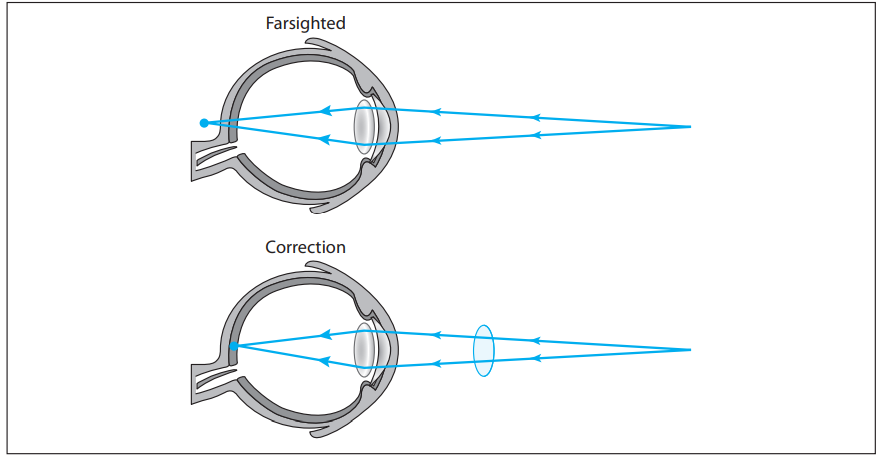Correction of hyperopia is accomplished with a converging lens tailored to characteristics unique to the person. The following table lists four different scenarios of a converging lens, with each scenario detailing the distance of an identical object placed in front of the same converging lens as well as the focal length of the lens.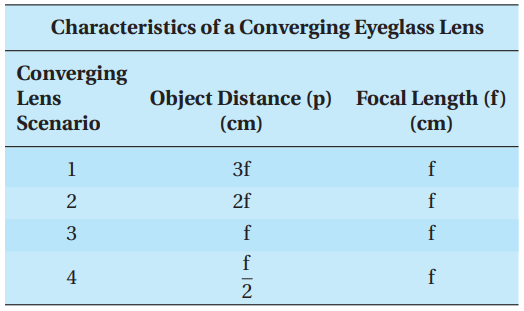Q15. Which converging lens scenario will NOT produce an image?

• A. Lens Scenario 1
• B. Lens Scenario 2
• C. Lens Scenario 3
• D. Lens Scenario 4

Q16. Which converging lens scenario will produce an image that is virtual and upright?

• A. Lens Scenario 1
• B. Lens Scenario 2
• C. Lens Scenario 3
• D. Lens Scenario 4

Q17. The image distance for Lens Scenario 2 is:

• A. 3f
• B. between 2f and 3f
• C. 2f
• D. f

Q18. The magnification of the image for Lens Scenario 1 indicates that, with respect to the object, the image:

• A. is reduced by 2.
• B. remains the same.
• C. is enlarged by 2.
• D. is enlarged by 3.

Q19. The focal length is related to the object distance and the image distance according to: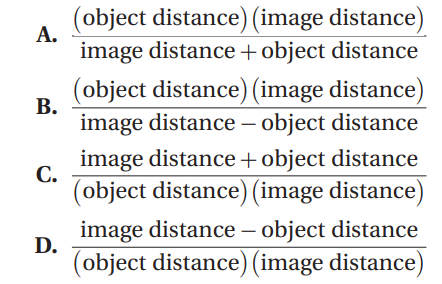Questions 20–22 are not associated with a passage.

Q20. Which of the following Fischer projections MOST accurately describes the boxed molecule?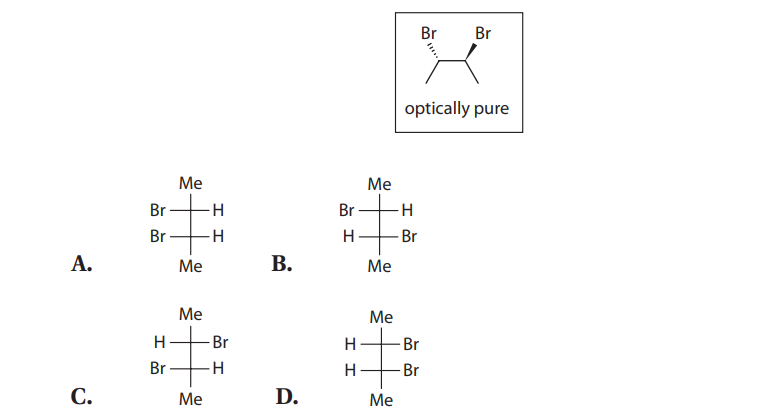Q21. For the following reaction, the most reasonable starting material is: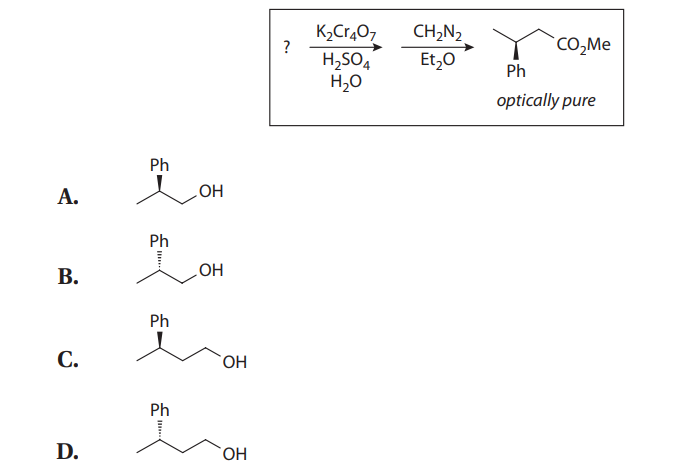Q22. For the following reaction, the most likely product is an:?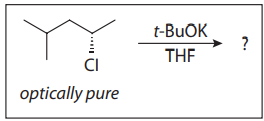• A. ether with optical activity
• B. ether without optical activity
• C. alkene with optical activity
• D. alkene without optical activity

Passage 4

An important class of oxidation-reduction reactions is called single displacement. In single displacement reactions, one element takes the place of another in a compound. The reactivity series for metals is a summary of the results of many single displacement experiments. A metal that is above another is more reactive and will take the place of the lower, less reactive metal in a compound.

For instance, a student places a sample of zinc metal into a solution of nickel (II) nitrate. The zinc reacts to displace the nickel ions as follows:

Zn (s) + Ni(NO3)2 (aq) → Ni (s) + Zn(NO3)2 (aq)

The reactivity series also predicts the reactivity of a metal with water. Only a few metals—the Group I metals and calcium, strontium, and barium from Group II— react with water at room temperature. This reaction is similar to a single displacement because the metal displaces the hydrogen in water. It is often called a hydrogen displacement reaction.

Dilute acid, usually hydrochloric acid, can be used to compare the reactivity of metals that do not react with water.

Experiment

A student reacts samples of copper, cobalt, and iron metal with dilute hydrochloric acid with the following results:

A general rule for the main group metals is that the reactivity of metals within a group increases going down the column, and the reactivity decreases going from left to right across a row. For transition metals, the reactivity does not change in a regular manner across a row but does decrease going down a column.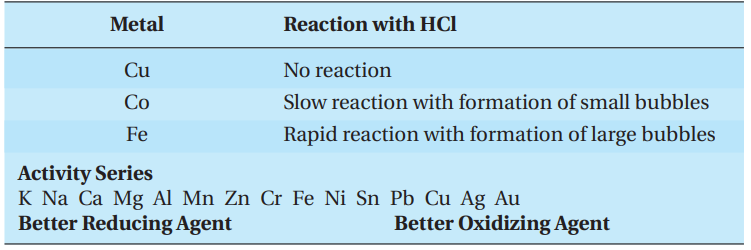Q23. Which of the following BEST represents the reaction of calcium with water?

• A. Ca (s) + H2O (l)→CaOH (s) + H (g )
• B. Ca (s) + H2O (l)→Ca(OH)2 (s) + H2 (g )
• C. Ca (s) + 2H2O (l)→Ca(OH)2 (s) + H2 (g )
• D. Ca+² (aq) + 2H2O (l)→Ca(OH)2 (aq) + H2 (g )

Q24. Which of the following is the first metal in the activity series that does NOT react with water?

• A. Mg
• B. Al
• C. Mn
• D. Zn

Q25. Where would cobalt be expected to fall in the activity series?

• A. between lead and copper
• B. to the left of iron
• C. between iron and copper
• D. to the right of copper

Q26. Which of the following statements is NOT true?

• A. Nickel will displace silver from silver (I) nitrate.
• B. Chromium reacts with dilute hydrochloric acid.
• C. Calcium will displace aluminum from aluminum nitrate.
• D. Tin will displace nickel from nickel (II) nitrate.

Q27. What is the electron configuration of chromium?

• A. [Ar]4s23d4
• B. [Ar]3d54s1
• C. [Ne]3d54s1
• D. [Ar]4s03d6

Questions 28–30 are not associated with a passage.

Q28. Which of the following metals is diamagnetic?

• A. calcium
• B. aluminum
• C. titanium
• D. copper

Q29. Iodine-131, a radioisotope typically employed in the diagnosis of thyroid disorders, has a mass number, A, of 131 and an atomic number, Z, of 53. The number of neutrons, N , of iodine-131 is:

• A. 131
• B. 78
• C. 53
• D. 25

Q30. Ten milliliters of blood is drawn for routine testing. Assuming the density of human blood is 1.06 g/cm³, the weight of the blood sample is:

• A. 0.1 N.
• B. 1.0 N.
• C. 10.0 N.
• D. 20.0 N.

Passage 5

The SN2 reaction is applicable to a wide variety of substrates, and it is impacted by many parameters. First, a good leaving group is required for SN2 to take place. Second, nucleophilic displacement is dependent upon a strong nucleophile; however, most potential nucleophiles exhibit both basic and nucleophilic character. As nucleophiles become more basic, the competing pathway of bimolecular elimination (E2) begins to predominate.

Two more parameters also exert influence on reactivity: the electrophilic center (i.e., primary, secondary, or tertiary) and the solvent (i.e., nonpolar, polar protic, or polar aprotic). A student mixes equimolar quantities of 2-chloropropane, 2-iodopropane, sodium methoxide (NaOMe), and sodium methyl thiolate (NaSMe) in a solvent of methanol, and observes both SN2 and E2 reactivity.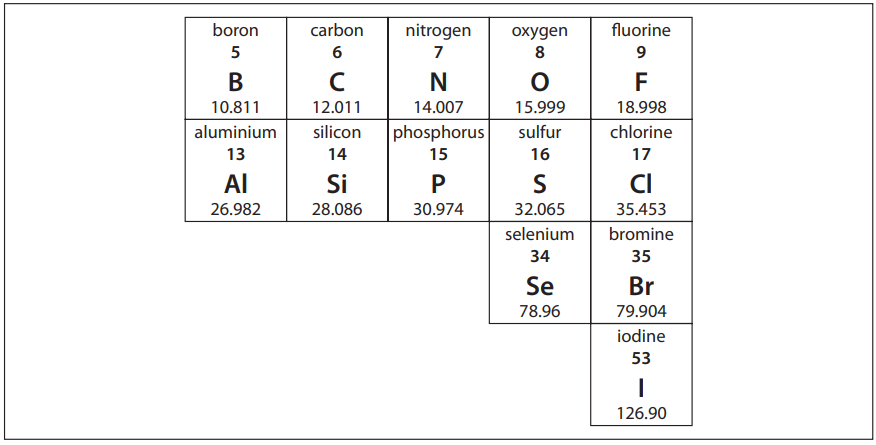Two more parameters also exert influence on reactivity: the electrophilic center (i.e., primary, secondary, or tertiary) and the solvent (i.e., nonpolar, polar protic, or polar aprotic). A student mixes equimolar quantities of 2-chloropropane, 2-iodopropane, sodium methoxide (NaOMe), and sodium methyl thiolate (NaSMe) in a solvent of methanol, and observes both SN2 and E2 reactivity.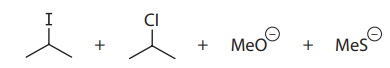Q31. In comparing methoxide (MeO) and methyl thiolate (MeS), methoxide is:

• A. more nucleophilic and more basic
• B. more nucleophilic and less basic
• C. less nucleophilic and more basic
• D. less nucleophilic and less basic

Q32. In comparing chloride (Cl) and iodide (I), chloride is:

• A. the better leaving group and the better nucleophile
• B. the better leaving group and the poorer nucleophile
• C. the poorer leaving group and the better nucleophile
• D. the poorer leaving group and the poorer nucleophile

Q33. The fastest SN2 reaction would occur between:

• A. methoxide and 2-iodopropane
• B. methoxide and 2-chloropropane
• C. methyl thiolate and 2-iodopropane
• D. methyl thiolate and 2-chloropropane

Q34. If temperature is increased,

• A. the rate of E2 increases; the rate of SN2 decreases
• B. the rate of SN2 increases; the rate of E2 decreases
• C. the rate of both reactions increases
• D. the rate of both reactions decreases

Q35. Which of the following solvents would provide for the fastest SN2 reaction?

• A. methanol
• B. dimethyl sulfoxide
• C. tetrahydrofuran
• D. diethyl ether

Passage VI

Cervical traction devices employ forces transmitted through a system of pulleys to correct deformities of the neck and spine by maintaining their proper alignment. The physics involved in these devices are based on Newton’s second law of motion and Atwood’s machine.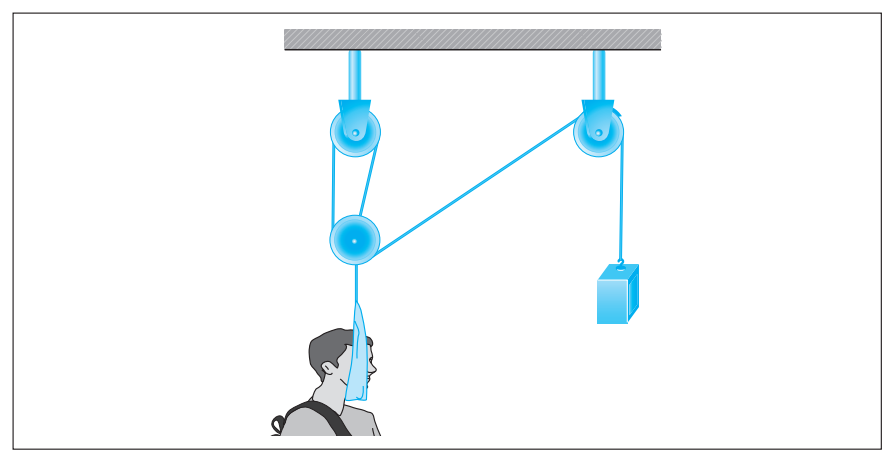Newton’s second law of motion states that a net force, Fnet, acting in a given direction on an object of mass, m, will result in an acceleration, a, of the object in that same direction, i.e.: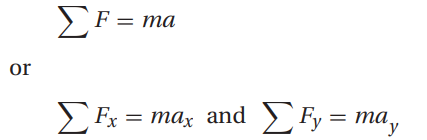Atwood’s machine, illustrated in the following figure, uses a block-and-pulley system to determine the acceleration of the system as well as other variables such as the falling distance of either mass and the tension in the string connecting the masses.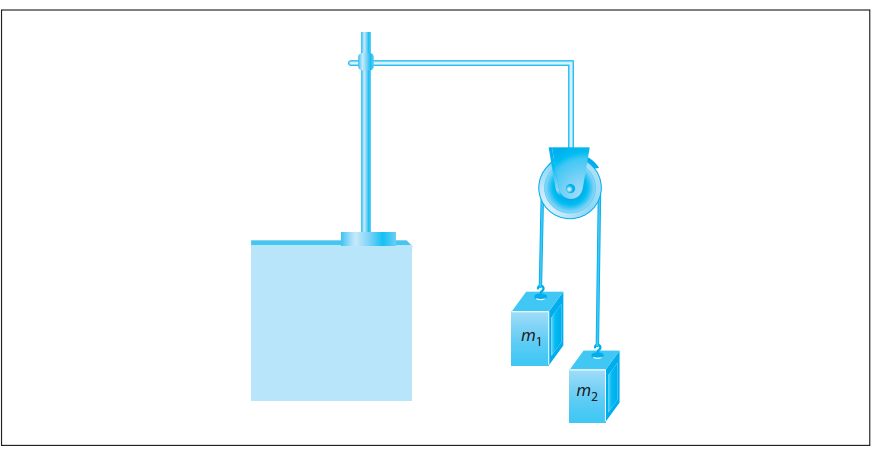In a typical experimental setup, the Atwood machine is assembled with a massless string around a pulley. Connected on either end of the string are two masses, m1 and m2, where m1 ≠ m2. The pulley is considered to be massless as well as frictionless. When the connected masses are released, both masses will accelerate at the same rate with the larger mass accelerating downward and the smaller mass accelerating upward.

Students were asked to conduct experiments using Atwood’s machine with a constant total mass of 300 g. They were given hanging weights in increments of 10 g and could place any number of weights on either side of Atwood’s machine as long as the total mass equaled 300 g.

Q36. For a typical Atwood’s machine setup, the free-body diagram of the side with m1 is: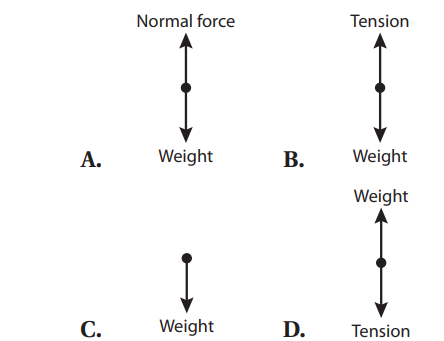Q37. A general expression for the acceleration of the system in Atwood’s machine is: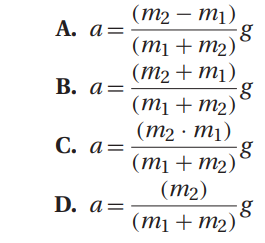Q38. A trial is conducted using the Atwood’s machine with m1 = 140 g and m2 = 160 g. The tension in the string is approximately:

• A. 0.8 N
• B. 1.2 N
• C. 1.5 N
• D. 1.9 N

Q39. Students applied masses to the Atwood’s machine setup such that m1 = 80 g and m2 = 220 g. The acceleration of the system is: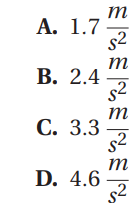Q40. In an experimental trial in which the students used masses m1 = 110 g and m2 = 190 g, the students determined the tension in the string to be:

• A. 1.4 N
• B. 1.9 N
• C. 2.8 N
• D. 3.3 N

Q41. An assumption of the calculation for the acceleration of the system of masses in Atwood’s machine is that the pulley is frictionless. If one uses a pulley with friction, the expression for the acceleration of the system now becomes: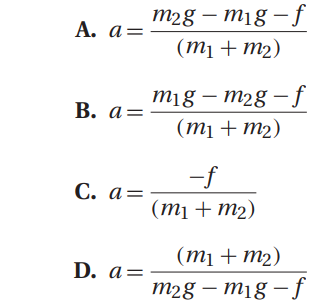Q42. In an experiment using a pulley with friction, the students collect data and create a graph of acceleration versus mass difference. From the graph, the students can determine the frictional force from the:

• A. x-variable
• B. y-variable
• C. slope
• D. y-intercept

Passage 7

The Jones oxidation is a classical methodology for the oxidation of secondary alcohols to ketones. The conditions involve the combination of potassium dichromate (K2Cr2O7), a shelf-stable crystalline solid, and aqueous sulfuric acid (H2SO4). This is simply a way to produce chromic acid (H2CrO4) in situ: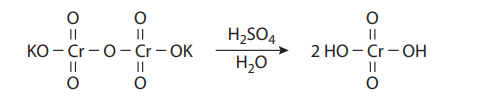Under the reaction conditions, chromic acid produces equilibrium concentrations of chromium trioxide (CrO3), which is the key oxidizing agent in the Jones oxidation: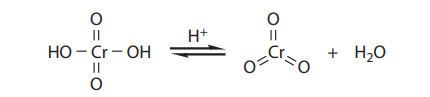Chromium trioxide reacts with alcohols to provide chromate esters, which proceeds as follows: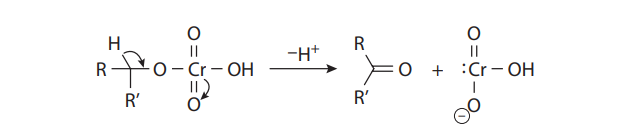With primary alcohols, however, the reaction does not stop at the aldehyde stage. In the presence of acid and water, the initially formed carbonyl compounds are in equilibrium with their hydrates:The hydrates can form chromate esters with one of the hydroxyl groups, just as the alcohol substrates do. When R´= H, this proton is easily lost to provide the carboxylic acid. Therefore, under Jones conditions, secondary alcohols yield ketones, but primary alcohols give carboxylic acids:If aldehydes are desired from primary alcohols, the oxidation can be carried out with pyridinium chlorochromate (PCC), a shelf-stable crystalline solid that also provides chromium trioxide: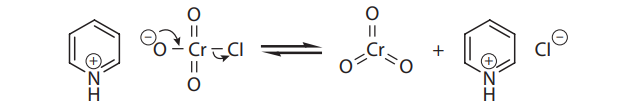Q43. Ketones are NOT oxidized to carboxylic acids under Jones conditions because:

• A. They do not form hydrates with water.
• B. Their hydrates cannot form chromate esters.
• C. The chromate esters of their hydrates cannot decompose by proton loss.
• D. The chromate ester decomposition is reversible.

Q44. Pyridinium chlorochromate (PCC) is selective for the production of aldehydes from primary alcohols because:

• A. It can be weighed out very accurately.
• B. It does not introduce water into the reaction.
• C. The pyridine prevents overoxidation.
• D. The environment is less acidic.

Q45. Consider the Jones oxidation of heptane-1,6-diol: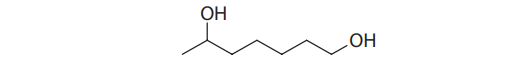The MOST likely product of this reaction would be a(n):

• A. hydroxy ketone
• B. hydroxy acid
• C. oxo aldehyde
• D. oxo acid

Q46. Consider the PCC oxidation of heptane-1,6-diol: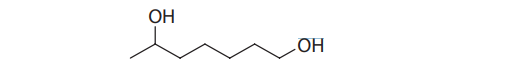The MOST likely product of this reaction would be a(n):

• A. hydroxy ketone
• B. hydroxy acid
• C. oxo aldehyde
• D. oxo acid

Q47. A tertiary alcohol is treated with the Jones reagent, and the reaction mixture is worked up and purified.

The MOST likely compound isolated is a(n):

• A. alcohol
• B. ketone
• C. aldehyde
• D. carboxylic acid

Passage 8

α-D-glucose and β-D-glucose are interconverted through a process known as mutarotation, which proceeds via a ring-opened intermediate, as shown: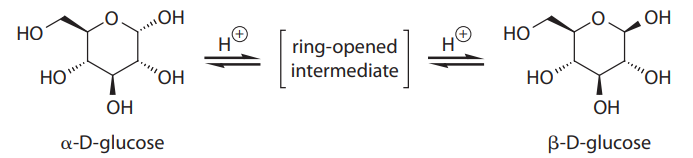In solution, α-D-glucose and β-D-glucose exist in a 9:16 ratio at equilibrium, but this is not what would be predicted from sterics alone. The discrepancy is largely due to the anomeric effect, which involves the interaction of the lone pairs on the ring oxygen with the C-1 hydroxy group.

Q48. The best term for describing the difference betweenα-D-glucose andβ-D-glucose is:

• A. enantiomers
• B. diastereomers
• C. regioisomers
• D. conformers

Q49. Aside from the multiple alcohols, the functional group found in both α-D-glucose and β-D-glucose is a(n):

• A. hemiacetal
• B. acetal
• C. aminal
• D. orthoester

Q50. Aside from the multiple alcohols, the functional group found in the ring-opened intermediate is a(n):

• A. ketone
• B. aldehyde
• C. acid
• D. ester

Q51. α-D-glucose and β-D-glucose are BEST described as:

• A. hexoses and furanoses
• B. pentoses and furanoses
• C. hexoses and pyranoses
• D. pentoses and pyranoses

Q52. The BEST rationale for the impact of the anomeric effect on the equilibrium of α-D-glucose and β-D-glucose is:

• A. It stabilizes α-D-glucose and destabilizes β-D-glucose.
• B. It destabilizes α-D-glucose and stabilizes β-D-glucose.
• C. It stabilizes both α-D-glucose and β-D-glucose.
• D. It destabilizes both α-D-glucose and β-D-glucose.

Passage 9

In the general chemistry laboratory, it is convenient to carry out most reactions in solution, usually aqueous solution. When solid ionic compounds are mixed together at room temperature, no reaction takes place. But if aqueous solutions of the solids are mixed, a reaction can take place. Common types of reactions in aqueous solution include acid-base reactions, precipitation reactions, and oxidation-reduction reactions.

Compounds have different solubilities in water, as shown in the following table: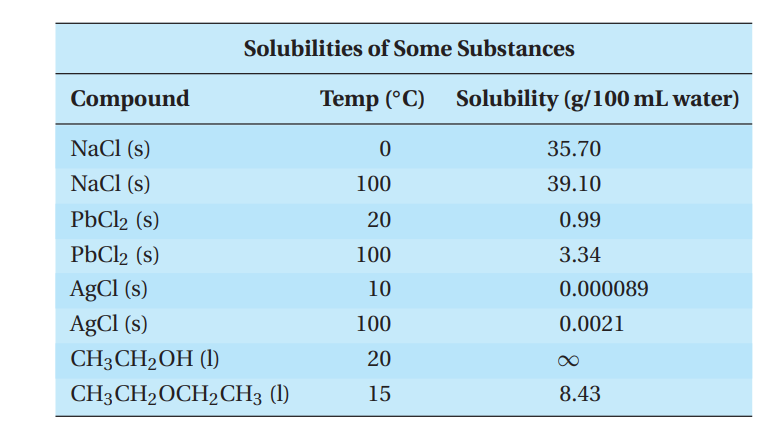Ions in water solution are called electrolytes, and a solution of electrolytes will conduct electricity. The ability to conduct electricity is called conductivity, and it is directly related to the concentration of ions in solution. Ionic compounds that are very soluble in water are strong electrolytes. Ionic compounds that are sparingly soluble in water are weak electrolytes. Covalent compounds can be strong, weak, or even nonelectrolytes. It depends on whether the substance can form ions in solution, and if so, the concentration of the ions.

A general rule for predicting whether a reaction will take place when two electrolytic solutions are mixed is that a reaction will take place if one or both possible products are either insoluble, weak, or nonelectrolyte.

Experiment

A student determines if each of a series of compounds was soluble or insoluble in water. The results are as follows.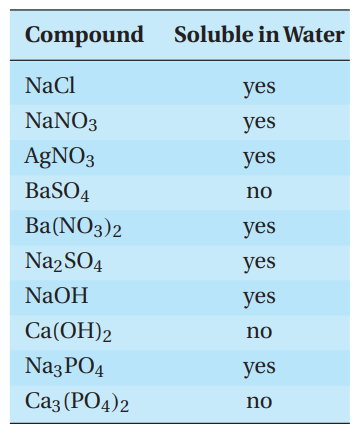Q53. What is the expected insoluble product when a solution of silver nitrate reacts with a solution of sodium chloride at room temperature?

• A. sodium nitrate
• B. silver nitrate
• C. silver chloride
• D. sodium chloride

Q54. A student prepares a saturated solution of sodium chloride at 100.0 ◦C. The student makes 250.0 mL of solution and then cools it to 0.0 ◦C. How many grams of NaCl will precipitate out when the solution is cooled to 0.0 ◦C?

• A. 89.3 g
• B. 97.8 g
• C. 8.6 g
• D. 3.4 g

Q55. What would happen to the conductivity of a mixture of two solutions that produces two insoluble products?

• A. It would remain the same.
• B. It would lessen somewhat.
• C. It would decrease greatly.
• D. It would increase greatly.

Q56. Which of the following statements can BEST be inferred from the experimental results?

• A. Sodium salts are always soluble.
• B. Group II salts are always soluble.
• C. Phosphates are usually insoluble.
• D. Sulfates are usually soluble.

Q57. Based on the experimental data, which of the following statements is true?

• A. Silver compounds are always insoluble.
• B. Phosphates are always insoluble.
• C. Sulfates are never soluble.
• D. You can improve the solubility of silver chloride with the addition of ammonia.

Questions 58 and 59 are not associated with a passage.

Q58. Which of the following shows a pair of substances that differ from the other three?